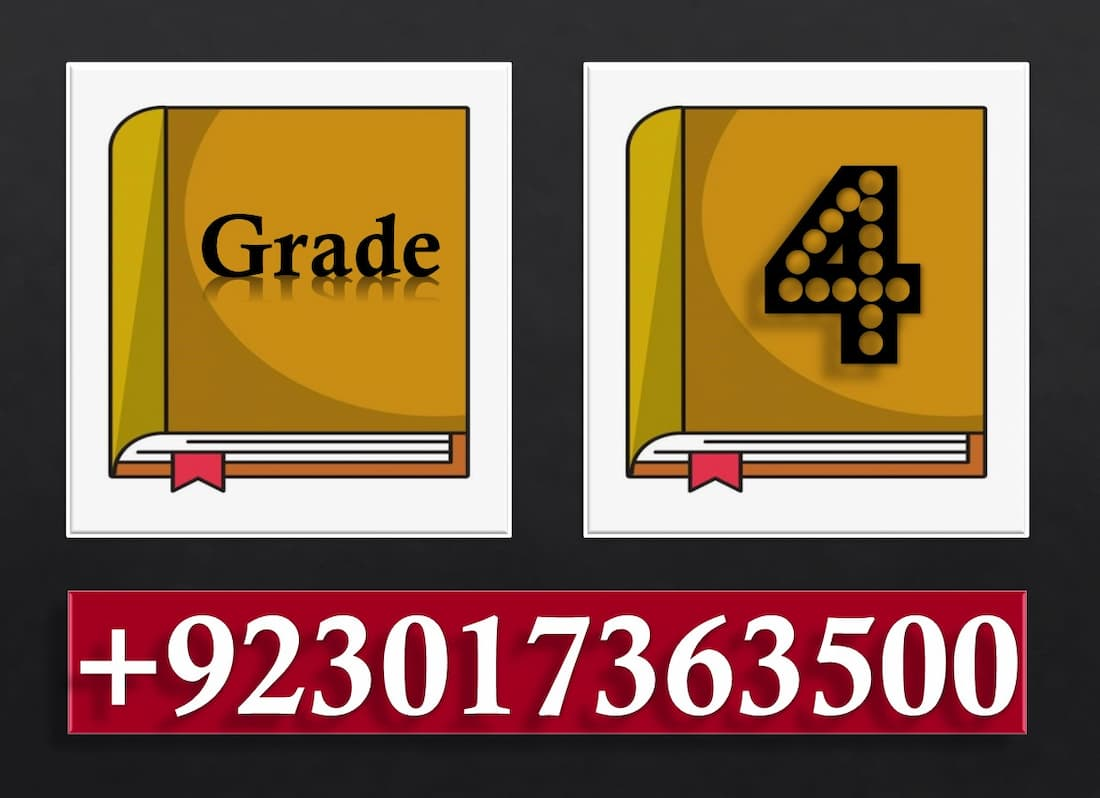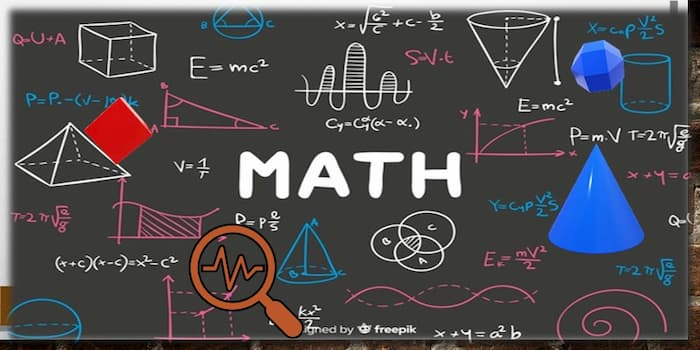Dear Students You know that Math is a fun. We believe that the best way to learn mathematics (Trigonometry Formula, Common Core Math, Math facts, Math practice Worksheets and Grade 4 math worksheets,) is to practice as many exercises as possible. That’s why many Printable 4th Grade Math worksheets have been created, separated by themes, so that each student can practice in the subjects where he / she feels the most difficulty.Subtraction Worksheets

This is the main page for the subtraction worksheets. Dear Students, All 4th   Grade Math Worksheets pdf free Printable download for Spaceship Math Subtraction worksheets, timed subtraction tests, multiple digit subtraction worksheets, simple borrowing, Subtraction with regrouping, subtracting decimals, Long Subtraction, Double Digit Subtraction   and regrouping worksheets, and math worksheets with mixed addition and subtraction problems

Multiplication Worksheets

Dear Students, here are multiplication worksheets for You. Put your fingers away, because this is the first math operation where memorization of the facts is a requirement. Dear Students, here multiplication Printable 4th Grade Math worksheets for Dad’s Eight Simple Rules for Mastering the Times Table, multiple digit multiplication, squares and other multiplication worksheet topics.  These multiplication Free Printable worksheets include answer keys and are instantly printable and ready classroom or

Division Worksheets

This is the main page for the division worksheets. This includes Shuttle Math Division worksheets Grade 4 , multiple digit division worksheets, square root worksheets, cube roots, mixed multiplication and division worksheets. These division free Printable 4th Grade Math worksheets are free for personal or classroom use.

Telling Analog Time

The Free Printable 4th Grade Math worksheets for telling analog clock time, including both reading time and drawing clock faces.   Dear Students, we will discuss here these topics e.g. Telling on an analog clock, analog clock Time and analog clock with Seconds

Analog Elapsed Time

Free Printable 4th Grade Math Worksheets comparing two analog clocks and determining how much time has elapsed between the two. (elapsed time number line, Finding elapsed time)